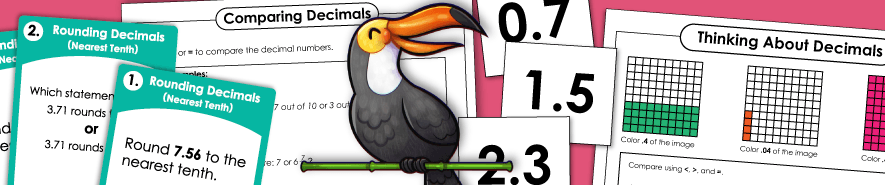# Decimal Printables## Understanding Decimals

Basic Decimals (Tenths and Hundredths)

Teach students to read, write, and recognize basic decimals. Includes worksheets with only tenths, only hundredths, only thousandths, as well as a mix of all three. Also includes fraction to decimal conversions.

Comparing and Ordering Decimals

Learn to order and compare the value of decimal numbers.

Rounding Decimals

Round decimals to the nearest tenth, hundredth, and/or thousandth.

Decimal Place Value: Tenths

On these worksheets, you'll find place value concepts applied to decimals. Most decimals are to the nearest thousandth.

Decimal Place Value: Hundredths

These place value worksheets include decimals with a hundredths place. Worksheets include: determining the values of digits, rounding, and ordering numbers.

Decimal Place Value: Thousandths

Here we have a variety of worksheets with decimals that have a thousandths place.

## Operations with Decimals

Practice adding and subtracting decimals numbers.

Multiplying Decimals

Practice multiply decimals by 1 and 2-digit numbers. Includes a variety of horizontal, vertical, and word problems.

Dividing Decimals

Practice dividing decimals with 1-digit and 2-digit divisors.

Percents

Give fractions or decimals, find the percentages.

Fractions

THis page has fraction place value activities, as well as fraction rounding and equivalent fractions. There are also addition, subtraction, multiplication, and division of fraction worksheets.